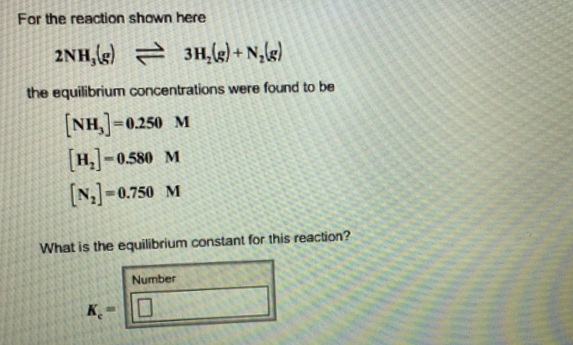# Problem: For the reaction shown here 2NH3(g) ⇌ 3H2(g) + N2(g)the equilibrium concentrations were found to be [NH3] = 0.250 M [H2] = 0.580 M [N2] = 0.750 M What is the equilibrium constant for this reaction?

🤓 Based on our data, we think this question is relevant for Professor Lakshminarayanan's class at CSUN.

###### FREE Expert Solution###### Problem Details

For the reaction shown here

2NH3(g) ⇌ 3H2(g) + N2(g)

the equilibrium concentrations were found to be

[NH3] = 0.250 M

[H2] = 0.580 M

[N2] = 0.750 M

What is the equilibrium constant for this reaction?